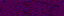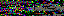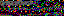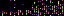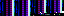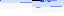```from pygame import display
from pygame.font import Font
from pygame.time import get_ticks, wait

from GameChild import GameChild

class Mainloop(GameChild):

def __init__(self, parent):
GameChild.__init__(self, parent)
self.overflow = 0
self.frame_count = 1
self.actual_frame_duration = 0
self.frames_this_second = 0
self.last_framerate_display = 0
self.init_framerate_display()
self.last_ticks = get_ticks()
self.stopping = False

config = self.get_configuration("display")
self.target_frame_duration = config["frame-duration"]
self.wait_duration = config["wait-duration"]
self.skip_frames = config["skip-frames"]
self.show_framerate = config["show-framerate"]
self.framerate_text_size = config["framerate-text-size"]
self.framerate_text_color = config["framerate-text-color"]
self.framerate_text_background = config["framerate-text-background"]
self.framerate_display_flag = config["framerate-display-flag"]

def init_framerate_display(self):
if self.framerate_display_active():
screen = self.get_screen()
self.last_framerate_count = 0
self.framerate_topright = screen.get_rect().topright
self.display_surface = screen
self.font = Font(None, self.framerate_text_size)
self.font.set_bold(True)
self.render_framerate()

def framerate_display_active(self):
return self.check_command_line(self.framerate_display_flag) or \
self.show_framerate

def render_framerate(self):
text = self.font.render(str(self.last_framerate_count), False,
self.framerate_text_color,
self.framerate_text_background)
rect = text.get_rect()
rect.topright = self.framerate_topright
self.framerate_text = text
self.framerate_text_rect = rect

def run(self):
while not self.stopping:
self.update_frame_duration()
self.update_overflow()
self.stopping = False

refresh = False
while self.frame_count > 0:
refresh = True
self.parent.frame()
if self.framerate_display_active():
self.update_framerate()
self.frame_count -= 1
if not self.skip_frames:
break
if refresh:
display.update()

def update_frame_duration(self):
last_ticks = self.last_ticks
actual_frame_duration = get_ticks() - last_ticks
last_ticks = get_ticks()
while actual_frame_duration < self.target_frame_duration:
wait(self.wait_duration)
actual_frame_duration += get_ticks() - last_ticks
last_ticks = get_ticks()
self.actual_frame_duration = actual_frame_duration
self.last_ticks = last_ticks

def update_overflow(self):
self.frame_count = 1
target_frame_duration = self.target_frame_duration
overflow = self.overflow
overflow += self.actual_frame_duration - target_frame_duration
while overflow > target_frame_duration:
self.frame_count += 1
overflow -= target_frame_duration
overflow = self.overflow

def update_framerate(self):
count = self.frames_this_second + 1
if get_ticks() - self.last_framerate_display > 1000:
if count != self.last_framerate_count:
self.last_framerate_count = count
self.render_framerate()
self.last_framerate_display = get_ticks()
count = 0
self.display_surface.blit(self.framerate_text, self.framerate_text_rect)
self.frames_this_second = count

def stop(self):
self.stopping = True
```
```from os import makedirs
from os.path import exists, join
from sys import exc_info
from time import strftime

from pygame import image

from GameChild import *
from Input import *

class ScreenGrabber(GameChild):

def __init__(self, game):
GameChild.__init__(self, game)
self.delegate = self.get_delegate()
self.subscribe(self.save_display)

config = self.get_configuration("screen-captures")
self.save_path = config["path"]
self.file_name_format = config["file-name-format"]
self.file_extension = config["file-extension"]

def save_display(self, event):
if self.delegate.compare(event, "capture-screen"):
directory = self.save_path
try:
if not exists(directory):
makedirs(directory)
name = self.build_name()
path = join(directory, name)
capture = image.save(self.get_screen(), path)
self.print_debug("Saved screen capture to %s" % (path))
except:
self.print_debug("Couldn't save screen capture to %s, %s" %\
(directory, exc_info()))

def build_name(self):
return "{0}.{1}".format(strftime(self.file_name_format),
self.file_extension)
```
```from math import sin, cos, atan2, radians, sqrt

def get_step(start, end, speed):
x0, y0 = start
x1, y1 = end
angle = atan2(x1 - x0, y1 - y0)
return speed * sin(angle), speed * cos(angle)

def get_endpoint(start, angle, magnitude):
"""clockwise, 0 is up"""
x0, y0 = start
dx, dy = get_delta(angle, magnitude)
return x0 + dx, y0 + dy

def get_delta(angle, magnitude):
return sin(angle) * magnitude, -cos(angle) * magnitude

def rotate_2d(point, center, angle, translate_angle=True):
if translate_angle:
x, y = point
cx, cy = center
return cos(angle) * (x - cx) - sin(angle) * (y - cy) + cx, \
sin(angle) * (x - cx) + cos(angle) * (y - cy) + cy

angle_step = 360.0 / count
points = []
current_angle = 0
for _ in xrange(count):
current_angle += angle_step
return points

if translate_angle:

def get_range_steps(start, end, count):
for ii in xrange(count):
yield start + (end - start) * ii / float(count - 1)

def get_distance(p0, p1):
return sqrt((p0 - p1) ** 2 + (p0 - p1) ** 2)
```
3.235.179.111
3.235.179.111
3.235.179.111

September 13, 2013

```from array import array
from time import sleep

import pygame
from pygame.mixer import Sound, get_init, pre_init

class Note(Sound):

def __init__(self, frequency, volume=.1):
self.frequency = frequency
Sound.__init__(self, self.build_samples())
self.set_volume(volume)

def build_samples(self):
period = int(round(get_init() / self.frequency))
samples = array("h",  * period)
amplitude = 2 ** (abs(get_init()) - 1) - 1
for time in xrange(period):
if time < period / 2:
samples[time] = amplitude
else:
samples[time] = -amplitude
return samples

if __name__ == "__main__":
pre_init(44100, -16, 1, 1024)
pygame.init()
Note(440).play(-1)
sleep(5)
```

This program generates and plays a 440 Hz tone for 5 seconds. It can be extended to generate the spectrum of notes with a frequency table or the frequency formula. Because the rewards in Send are idealized ocean waves, they can also be represented as tones. Each level has a tone in its goal and a tone based on where the player's disc lands. Both play at the end of a level, sounding harmonic for a close shot and discordant for a near miss. The game can dynamically create these tones using the program as a basis.

I'm also building an algorithmically generated song: Silk Routes (Scissored). Here is an example of how it sounds so far: